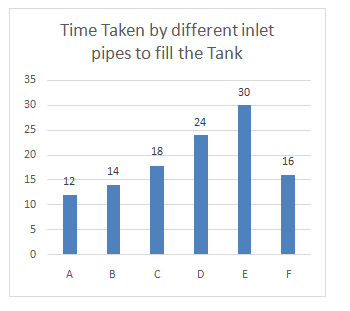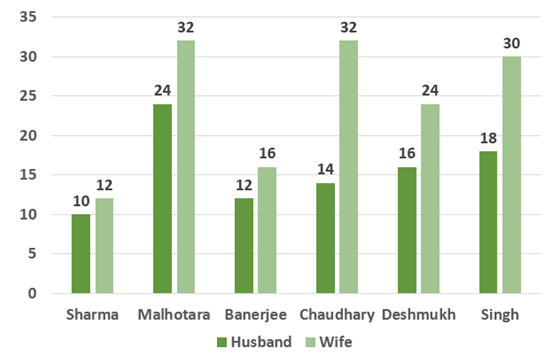# Quantitative Aptitude Questions (Data Interpretation) for SBI Clerk / IDBI Executive 2018 Day-68

Dear Readers, SBI is conducting Online preliminary Examination for the recruitment of Clerical Cadre. preliminary Examination of SBI Clerk was scheduled from June 2018. To enrich your preparation here we have providing new series of Data Interpretation – Quantitative Aptitude Questions. Candidates those who are appearing in SBI Clerk Prelims and IDBI Executive Exams can practice these Quantitative Aptitude average questions daily and make your preparation effective.

[WpProQuiz 1947]

Click “Start Quiz” to attend these Questions and view Solutions

Directions (Q. 1-5) Read the following information carefully and answer the following questions carefully.1. Inlet pipes A, B and outlet pipe S took 10 hours to fill the empty tank then find how much time Pipe S will take to empty a full tank?

a) 24(1/9)

b) 18(7/13)

c) 18(6/23)

d) 1(2/7)

e) 4(8/9)

2. Inlet pipe E and outlet pipe Q can fill the empty tank in 42 hours then how much time is required for inlet pipe C if it is opened with outlet Q to fill the tank?

a) 24(1/9)

b) 18(7/13)

c) 15(3/4)

d) 21(21/29)

e) 4(8/9)

3. Inlet pipes B, C and D were opened to fill tank so after how much time pipe C should be closed so that the tank gets filled in 8 hours?

a) 24(1/9)

b) 18(7/13)

c) 1(5/7)

d) 1(2/7)

e) 4(8/9)

1. Pipe F was opened alternatively with pipe T for one hour starting with F then find how much time will be required to fill the empty tank, if pipe F is three times efficeint as pipe T

a) 25

b) 18

c) 15

d) 24

e) 48

5. The time taken by inlet pipe D and outlet pipe P to fill the tank is 2 hours less than time taken by inlet pipe C and outlet S to fill the tank then find the time required by outlet pipe S to empty the full tank if the outlet pipe P can empty the full tank in 40 hours?

a) 24(1/9)

b) 25(4/11)

c) 15(3/4)

d) 1(2/7)

e) 4(8/9)

Directions (Q. 6 – 10): Study the following information carefully and answer the questions given below:

The following bar graph represents number of days taken by husbands and wives of six different families to complete a piece of work.1. If the husband of Sharma, Chaudhary and Singh family works together, in how many days the work will be completed?

a) 4(54/143) days

b) 4(58/143) days

c) 3(58/143) days

d) 4(58/141) days

e) None of these

1. Time taken by Malhotara family to complete the work is what percent of the time taken by Banerjee family to complete the work?

a) 200%

b) 220%

c) 280%

d) 100%

e) None of these

1. If Banerjee family and Chaudhary family works together, in how many days the work will get complete?

a) 5(4/167) days

b) 4(4/167) days

c) 3(4/167) days

d) 4(3/167) days

e) None of these

1. If the husband of Chaudhary family worked for 3 days and then left, in how many days Deshmukh family can complete the remaining work?

a) 7(19/35) days

b) 5(19/35) days

c) 3(19/35) days

d) 5(19/35) days

e) None of these

1. Find the time required by wife of all the families to complete the work.

a) 3(7/17) days

b) 3(9/17) days

c) 3(7/17) days

d) 4(9/17) days

e) None of these

Directions (Q. 1-5)

Total units of work= 420

A’s one hour work= 35 units

B’s one hour work= 30 units

A+B+S= 42 units

Units of work done by S in one hour= 65- 42= 23 units

Time required by S to empty the tank= 420/23= 18(6/23) hours.

Total units of work= 210 units

E’s one hour work= 7 units

Q’s and E’s one hour work= 5 units

Work done by Q in an hour= 7-5= 2 units

Time required by Q to empty the tank= 210/2= 105 hours.

Total units of work= 630

Q’s one work= 6 units

C’s one hour work= 35 units

Q’s and C’s one hour work= 35 – 6 = 29 units

Time required= 630/29= 21(21/29) hours.

Total units of work= 504

B’s one hour work= 36 units

C’s one hours work= 28 units

D’s one hour work= 21 units

Work done by B and D in 8 hours= (36+21)*8= 456 units

Units of work remaining= 504-456= 48 units

Time after which pipe C should be closed= 48/28= 1(5/7) hours.

Efficiency ratio of F and T = 3: 1

Time ratio of F and T = 1: 3

T takes 48 days to complete the whole work

Total units of work= 48 units

F’s one hour work= 3 units

T’s one hour work= 1 units

Work done by pipe F and T in 2 hours= 3+1= 4 units

Work done in 24 hours= 4*12= 48 units

So the time required to fill the tank= 24 hours.

Total units of work= 120 units

D’s one hour work= 5 units

P’s one hour work= 3 units

Work done by D and P in one hour= 5-3= 2 units

Time taken by D and P to fill the tank = 120/2= 60 hours

Time taken by C and S to fill the tank= 62 hours

Time taken by C to fill the tank = 18 hours

Total units of work= 558 units (LCM of 18 and 62)

Work done by pipe C = 31 units

Work done by pipe C and S= 9 units

Work done by pipe S= 31-9= 22 units

Time required by pipe S to fill the tank= 558/22= 25(4/11) hours.

Directions (Q. 6 – 10):

Let the work will be completed in x days.

X (1/10 + 1/14 + 1/18) = 1

=> x (63 + 45 + 35)/630 = 1

=> x = 630/143 = 4(58/143) days

Let the time taken by Malhotara family to complete the work = x days

Let the time taken by Banerjee family to complete the work = y days

X (1/24 + 1/32) = 1

=> x(4 + 3)/96 = 1

=> x = 96/7

Y(1/12 + 1/16) = 1

=> y(4 + 3)/48 = 1

=> y = 48/7

Required percentage = [(96/7)/(48/7)] x 100 = 200%

Let the work will get complete in x days.

x(1/12 + 1/16 + 1/14 + 1/32) = 1

=> x(56 + 42 + 48 + 21)/672 = 1

=> x = 672/167 days = 4(4/167) days

Let Deshmukh family can complete the remaining work in x days.

3/14 + x (1/16 + 1/24) = 1

=> x (3 + 2)/48 = 11/14

=> x = 264/35 days = 7(19/35) days

Let the time taken by wife of all the families to complete the work = x days

X (1/12 + 1/32 + 1/16 + 1/32 + 1/24 + 1/30) = 1

=> x (40 + 15 + 30 + 15 + 20 + 16)/480 = 1

=> x = 480/136

=> x = 60/17 = 3(9/17) days

Daily Practice Test Schedule | Good Luck

 Topic Daily Publishing Time Daily News Papers & Editorials 8.00 AM Current Affairs Quiz 9.00 AM Logical Reasoning 10.00 AM Quantitative Aptitude “20-20” 11.00 AM Vocabulary (Based on The Hindu) 12.00 PM Static GK Quiz 1.00 PM English Language “20-20” 2.00 PM Banking Awareness Quiz 3.00 PM Reasoning Puzzles & Seating 4.00 PM Daily Current Affairs Updates 5.00 PM Data Interpretation / Application Sums (Topic Wise) 6.00 PM Reasoning Ability “20-20” 7.00 PM English Language (New Pattern Questions) 8.00 PM General / Financial Awareness Quiz 9.00 PM